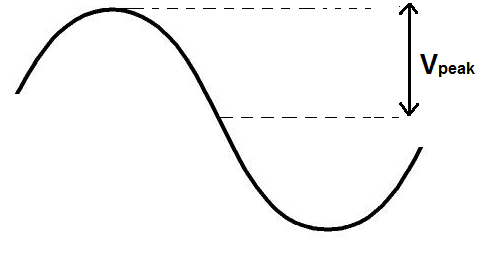﻿ Peak Voltage (Vp) Calculator ﻿# Peak Voltage (VP) CalculatorsV

0.0000V

V

0.0000V

### Average to Peak Voltage Calculator

V

0.0000V

The Peak Voltage calculator calculates the peak Voltage value from either the peak-to-peak voltage, the RMS voltage, or the average voltage. It calculates the peak Voltage based on the above formulas for each respectively.

To compute VP from the peak-to-peak voltage, the peak-to-peak voltage is multiplied by 0.5.

To compute VP from the RMS voltage, the RMS voltage is multiplied by 1.414.

To compute VPA user enters the voltage, V and the result will automatically be calculated and shown. The peak voltage result which is displayed above is in unit volts (V).

The peak voltage represents the voltage from the on the 0 degree vertical point to the peak of the waveform. It is used often to represent the amplitude of a voltage waveform. However, since there are so many other ways of specifying a voltage, including RMS voltage and average voltage, it should be specified as a peak value, such as 5Vpeak.

Related Resources

﻿